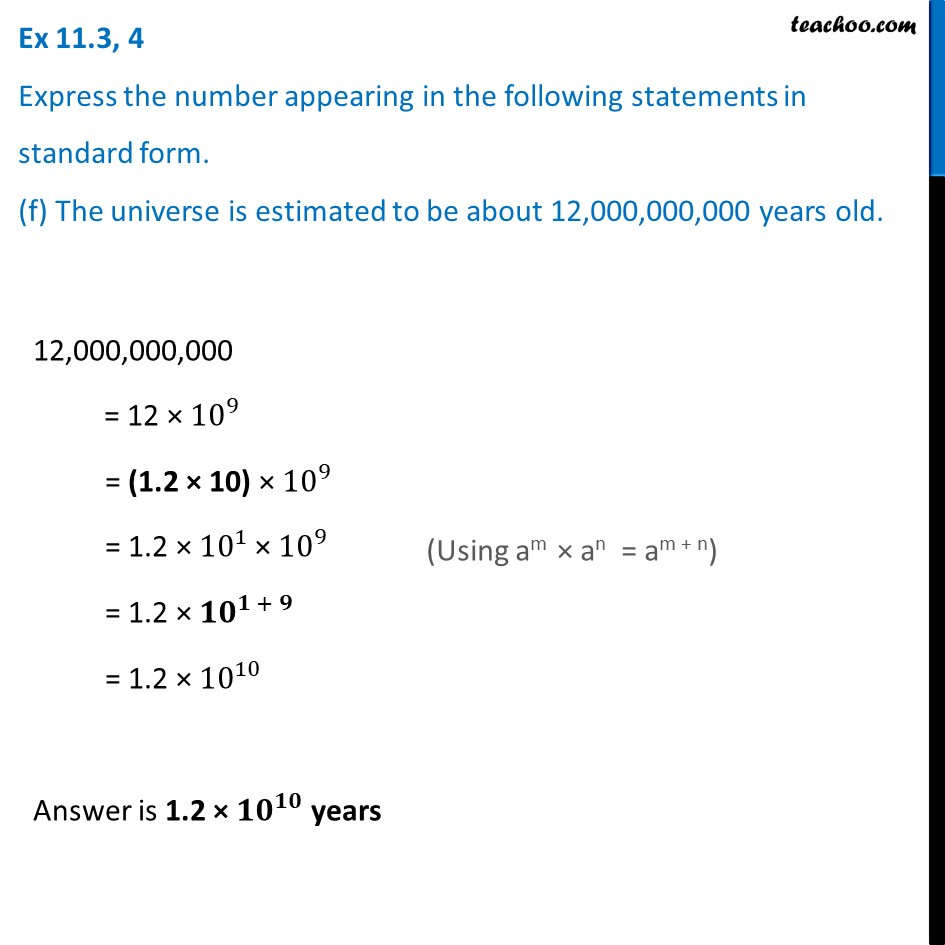Ex 11.3

Chapter 11 Class 7 Exponents and Powers
Serial order wiseLearn in your speed, with individual attention - Teachoo Maths 1-on-1 Class

### Transcript

Ex 11.3, 4 Express the number appearing in the following statements in standard form. (f) The universe is estimated to be about 12,000,000,000 years old. 12,000,000,000 = 12 × 〖10〗^9 = (1.2 × 10) × 〖10〗^9 = 1.2 × 〖10〗^1 × 〖10〗^9 = 1.2 × 〖10〗^(1 + 9) = 1.2 × 〖10〗^10 Answer is 1.2 × 〖𝟏𝟎〗^𝟏𝟎 years (Using am × an = am + n)